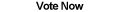Home Articles Components Code Snippets Links

C# Code Snippets# C# Fraction Structure

Using a structure we can represent fractions in C#.NET. Operation overloading allows developers to do common math operations with the fractions.

Platform: .NET Framework 2.0

```public struct Fraction
{
public int Numerator;
public int Denominator;
public static Fraction Zero = new Fraction(0, 0);

public Fraction(int numerator, int denominator)
{
this.Numerator = numerator;
this.Denominator = denominator;

//If denominator negative...
if (this.Denominator < 0)
{
//...move the negative up to the numerator
this.Numerator = -this.Numerator;
this.Denominator = -this.Denominator;
}
}

public Fraction(int numerator, Fraction denominator)
{
//divide the numerator by the denominator fraction
this = new Fraction(numerator, 1) / denominator;
}

public Fraction(Fraction numerator, int denominator)
{
//multiply the numerator fraction by 1 over the denominator
this = numerator * new Fraction(1, denominator);
}

public Fraction(Fraction fraction)
{
this.Numerator = fraction.Numerator;
this.Denominator = fraction.Denominator;
}

private static int getGCD(int a, int b)
{
//Drop negative signs
a = Math.Abs(a);
b = Math.Abs(b);

//Return the greatest common denominator between two integers
while (a != 0 && b != 0)
{
if (a > b)
a %= b;
else
b %= a;
}

if (a == 0)
return b;
else
return a;
}

private static int getLCD(int a, int b)
{
//Return the Least Common Denominator between two integers
return (a * b) / getGCD(a, b);
}

public Fraction ToDenominator(int targetDenominator)
{
//Multiply the fraction by a factor to make the denominator
//match the target denominator
Fraction modifiedFraction = this;

//Cannot reduce to smaller denominators
if (targetDenominator < this.Denominator)
return modifiedFraction;

//The target denominator must be a factor of the current denominator
if (targetDenominator % this.Denominator != 0)
return modifiedFraction;

if (this.Denominator != targetDenominator)
{
int factor = targetDenominator / this.Denominator;
modifiedFraction.Denominator = targetDenominator;
modifiedFraction.Numerator *= factor;
}

return modifiedFraction;
}

public Fraction GetReduced()
{
//Reduce the fraction to lowest terms
Fraction modifiedFraction = this;

//While the numerator and denominator share a greatest common denominator,
//keep dividing both by it
int gcd = 0;
while (Math.Abs(gcd = getGCD(modifiedFraction.Numerator, modifiedFraction.Denominator)) != 1)
{
modifiedFraction.Numerator /= gcd;
modifiedFraction.Denominator /= gcd;
}

//Make sure only a single negative sign is on the numerator
if (modifiedFraction.Denominator < 0)
{
modifiedFraction.Numerator = -this.Numerator;
modifiedFraction.Denominator = -this.Denominator;
}

return modifiedFraction;
}

public Fraction GetReciprocal()
{
//Flip the numerator and the denominator
return new Fraction(this.Denominator, this.Numerator);
}

public static Fraction operator +(Fraction fraction1, Fraction fraction2)
{
//Check if either fraction is zero
if (fraction1.Denominator == 0)
return fraction2;
else if (fraction2.Denominator == 0)
return fraction1;

//Get Least Common Denominator
int lcd = getLCD(fraction1.Denominator, fraction2.Denominator);

//Transform the fractions
fraction1 = fraction1.ToDenominator(lcd);
fraction2 = fraction2.ToDenominator(lcd);

//Return sum
return new Fraction(fraction1.Numerator + fraction2.Numerator, lcd).GetReduced();
}

public static Fraction operator -(Fraction fraction1, Fraction fraction2)
{
//Get Least Common Denominator
int lcd = getLCD(fraction1.Denominator, fraction2.Denominator);

//Transform the fractions
fraction1 = fraction1.ToDenominator(lcd);
fraction2 = fraction2.ToDenominator(lcd);

//Return difference
return new Fraction(fraction1.Numerator - fraction2.Numerator, lcd).GetReduced();
}

public static Fraction operator *(Fraction fraction1, Fraction fraction2)
{
int numerator = fraction1.Numerator * fraction2.Numerator;
int denomenator = fraction1.Denominator * fraction2.Denominator;

return new Fraction(numerator, denomenator).GetReduced();
}

public static Fraction operator /(Fraction fraction1, Fraction fraction2)
{
return new Fraction(fraction1 * fraction2.GetReciprocal()).GetReduced();
}

public double ToDouble()
{
return (double)this.Numerator / this.Denominator;
}

public override string ToString()
{
return Numerator + "/" + Denominator;
}
}
```

Back to C# Code Snippet List